Function Repository Resource:

# SophieGermainPrimeQ

Determine whether a number is a Sophie Germain prime

Contributed by: Arnoud Buzing
 ResourceFunction["SophieGermainPrimeQ"][p] gives True if p is a Sophie Germain prime and False otherwise.

## Details and Options

A Sophie Germain prime is a prime number q such that 2q+1 is also prime. Here, 2q+1 is referred as a safe prime.

## Examples

### Basic Examples (3)

11 is a Sophie Germain prime, because both 11 and 23=2·11+1 are primes:

 In:=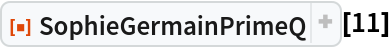Out=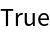7 is not a Sophie Germain prime, because 15=2·7+1 is not prime:

 In:=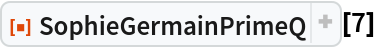Out=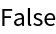Even though 29=2·14+1 is a prime number, 14 is not a Sophie Germain prime because it is not prime itself:

 In:=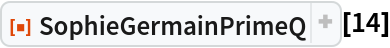Out=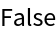Arnoud Buzing

## Version History

• 1.0.0 – 29 April 2020